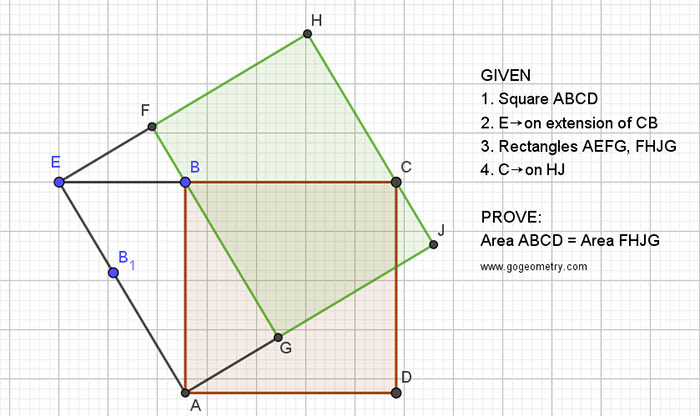## Friday, April 3, 2020

### Geometry Problem 1467: Square, Rectangle, Triangle, Area

Geometry Problem. Post your solution in the comment box below.
Level: Mathematics Education, High School, Honors Geometry, College.

Details: Click on the figure below.1.From sim triangles BC/GJ = EB/AG & EB/EA = AG/AB
=> BC/GJ = EA/AB

2.NOte that Triangles ABE and EHC are similar ( case AA)
so AB/AE=FH/BC => AB.BC=AE.FH
and area (ABCD)= area(FHJG)

3.AB=s, EB=a, EF=a', FH= s'
EA=d=sqrt(s²+a²)=FG
a':a= s:d => a'=as/d
s':a'=s:a => s'=s/a*as/d=s²/d
=> FH*FG=s'*d = s²